Open In App
Related Articles
• CBSE Class 12 Maths Notes
• NCERT Solutions for Class 12 Maths
• RD Sharma Class 12 Solutions for Maths

# Functions Defined by Integrals

While thinking about functions, we always imagine that a function is a mathematical machine that gives us an output for any input we give. It is usually thought of in terms of mathematical expressions like squares, exponential and trigonometric function, etc. It is also possible to define the functions in terms of definite integrals. These are similar to the area functions, which allow us to calculate the area under any curve given the limits for the definite integral. Let’s see how to define them and their properties in detail.

### Functions Defined by Definite Integrals

New functions can be defined with the help of integrals. Let’s say we are given a function f(x), then the area function is defined as the integral of the given function within some boundaries. Since there are boundaries within which we have to calculate the area, we have to use definite integrals for defining such functions. So, functions defined by integrals are given as,

F(x) =Let’s take an example of such function, assume

f(x) =if 0 ≤ x ≤ 5

The graph of the function will look roughly like this,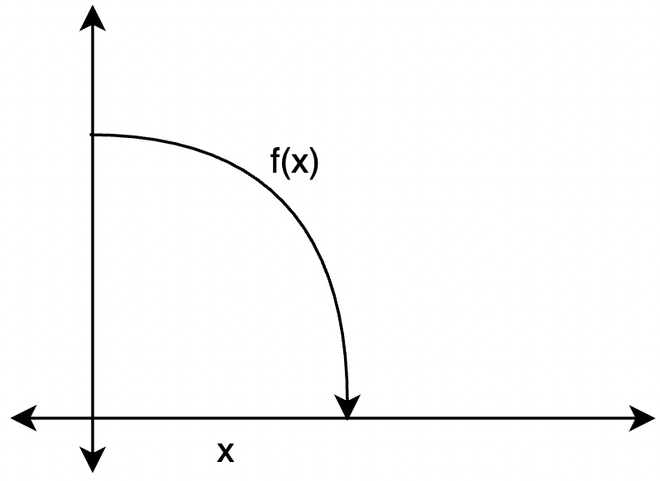Our goal is to find the function that gives the area under this curve. For a given particular value “x”, we calculate the integral from 0 to x.

F(x) =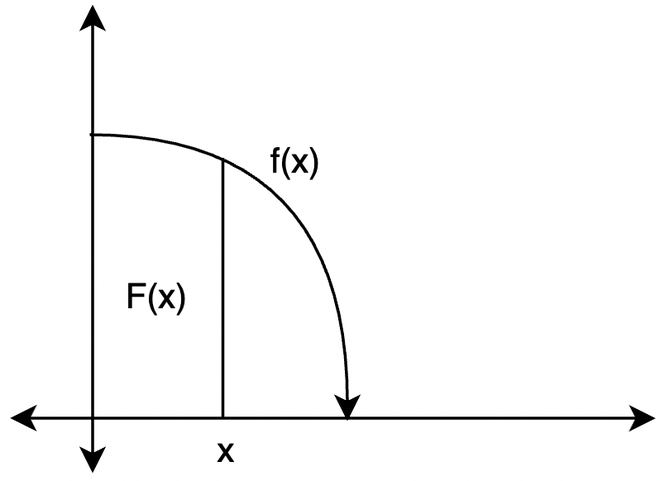What does this function mean? Since this function is the area under the graph of f, F(x) is basically the area under the curve from t = 0 to t = x. The figure below represents this area function from t = 0 to t = x.

### General properties of such functions

These properties allow us to draw the graph of such functions or simplify the calculation in cases where it is not easy to calculate the expressions for the functions:

1. Function F(x) is continuous where it is defined. This property comes from the fundamental theorem of calculus.
2. At x = a, value of the function F(a) = 0. This property comes from the properties of the definite integral.
3. F'(x) = f(x).
4. F”(x) = f'(x)
5. If a =0 and f(x) is even, then F(x) is odd.
6. If f(x) is odd, then F(x) is even.

### Natural Logarithm

Natural Logarithm is an example of the functions which are defined as functions of the integrals. For x > 0, natural logarithm is defined as,We can draw its curve using the properties mentioned above, calculating the x-intercept.

F(x) = ln(x) = 0 =This expression will be zero at x = 1. Thus, ln(x) = 0 at x = 1.

F'(x) =, F”(x) =Since F'(x) is always greater than zero for all the positive values of the input, the function is always increasing. Notice that with similar logic, we can say F”(x) < 0 for all the values of x greater than zero.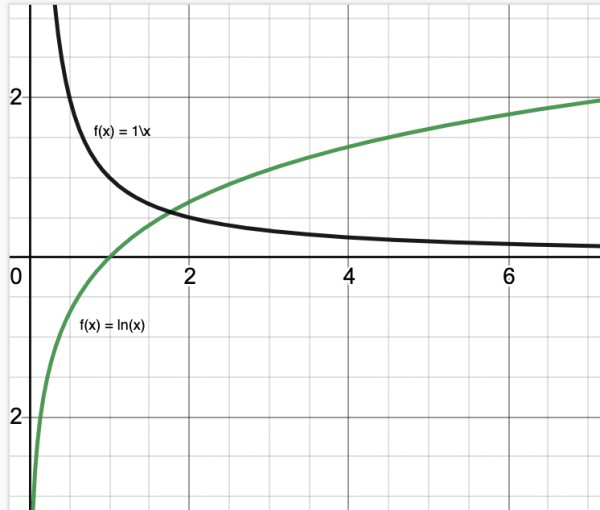### Error Function

For all real values of x, the error function is defined by,Let’s sketch graph for this function too,

It’s x intercept is given at erf(x) = 0, which is at x =0. Thus graph of this function passes through origin.

F(0) = 0, F'(x) =, F”(x) =F(x) will be odd since}is even. The graph of this function looks like,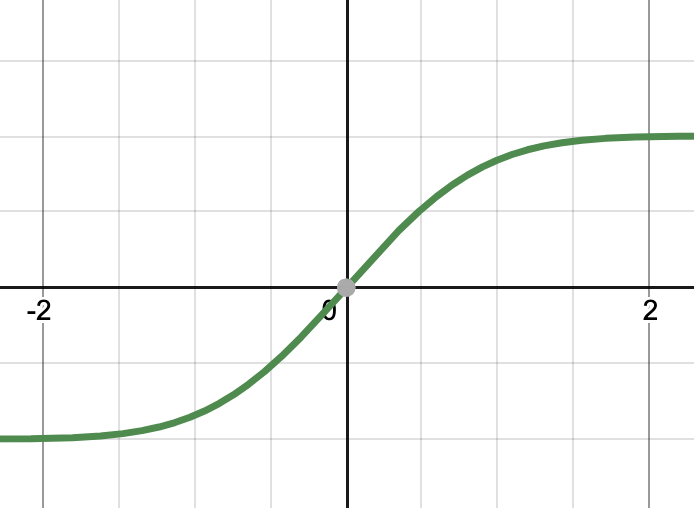### Sample Problems

Question 1: Given F(x) =. Prove that F'(x) = f(x) and F”(x) = f'(x).

Solution:

F(x) =⇒ F'(x) = f(x)

Differentiating it again,

F”(x) = f'(x)

Question 2: Given the F(x) =. Find the value of F(2).

Solution:

F(x) =.

at x = 2

F(x) =⇒ F(x) =⇒ F(x) =⇒ F(x) =Question 3: Given the F(x) =. Find the value of F(4).

Solution:

F(x) =.

at x = 4

F(4) =⇒ F(4) =⇒ F(4) =⇒ F(4) = 8

Question 4: Find the value of the given function g(x) at x = 3.

f(x) =g(x) =Solution:

The graph for the function f(x) is given by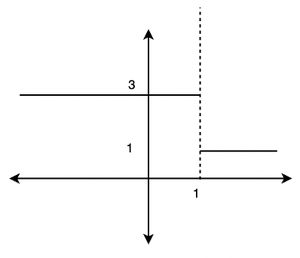We need to calculate the value of g(x) =g(3) =⇒ g(3)=⇒ g(3) =⇒ g(3) =⇒ g(3) = 3 + 1

⇒ g(3) = 4

Question 5: Find the value of the given function at x = 1.

f(x) =g(x) =Solution:

The graph for the function f(x) is given by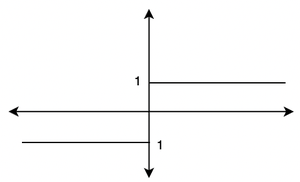We need to calculate the value of g(x) =g(1) =⇒ g(1)=⇒ g(1) =⇒ g(1) =⇒ g(1) =2

Question 6: Calculate the value of the given function f(x) at x = 1.

f(x) =Solution:

This integration can be solved by applying the by parts formula for integration.

We know thatApplying by parts integration to the integral

f(x) =⇒f(x) =f(1) = -1

Question 7: Calculate the value of the given function f(x) at x = 5.

f(x) =Solution:

This integration can be solved applying the by parts formula for integration.

We know thatApplying by parts integration to the integral

f(x) =⇒f(x) =f(1) = -1

Question 8: Calculate the value of the given function x = 2,

g(x) = f(x) + f(-x)

Where f(x) =Solution:

g(x) = f(x) + f(-x)

We know that f(x) is the error function.

Now by the properties mentioned above, sinceis an even function. The integral function must be an odd function.

That is, f(x) =is an odd function.

Since f(x) is an odd function, f(-x) = -f(x).

g(x) = f(x) + f(-x)

⇒ g(x) = f(x) – f(x)

⇒ g(x)= 0

Thus, g(2) = 0